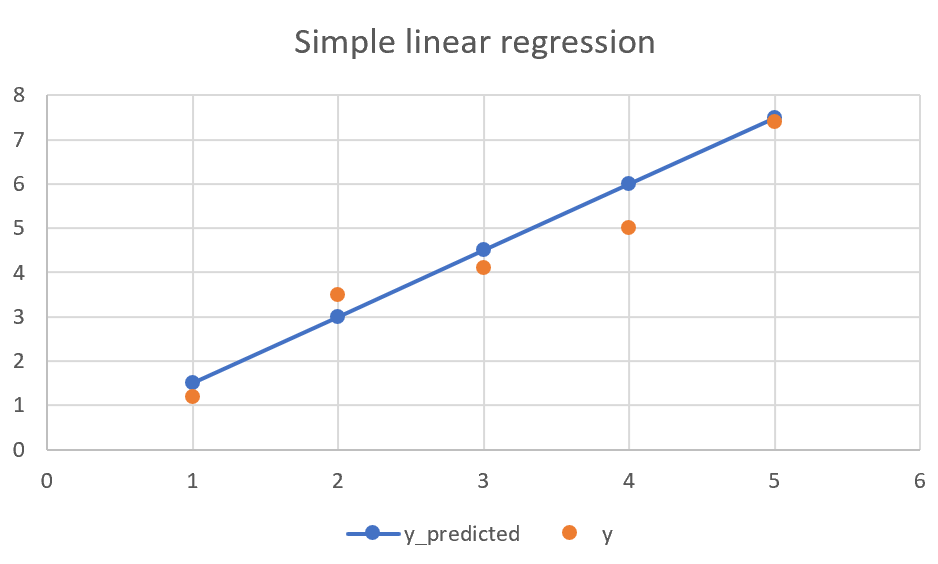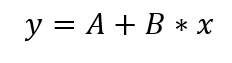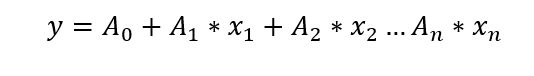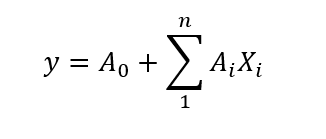# Multiple linear regression intuition

Subscribe to my newsletter and never miss my upcoming articles

After simple linear regression (SLR) logical next learning step is multiple linear regression (MLR). To better explain what MLR is, let's start with a reminder of what SLR is.

## Simple linear regression

SLR is a statistical method where the predicted value depends linearly on one input value. That means that mathematically we can describe it by simple linear functions and visualize it with a line.The formula for this would be:This is just a reminder of what SLR is. But for more information on it you can look at my previous post on it.

## Multiple linear regression

Now that you are reminded of what simple linear regression, we can move onto multiple linear regression. MLR is the same thing but with more than one input variable. Here is how it looks in a mathematical equation.## But what are all those variables?

Depending on where you look, all variables can have different names, but I’ll try to keep it simple with other commonly used terms.

y – the value we want to predict/dependent variable/predicted value Xi – features / independent variable / expanatory variable / observed variable Ai – the coefficient for feature

So simplified, we are predicting what value of y will be depending on features Xi, and with coefficients Ai we are deciding how much each feature is affecting the predicted value.

## More correct mathematical modelIn translation, predicted value y is the sum of all features multiplied with their coefficients, summed with base coefficient A0.

## Real-world example:

This example won’t show implementation. Some implementation examples you can find in my Github repository that follows Udemy Machine learning A-Z course.

Imagine we want to predict the salary of the employee should have. Input variables we have could be number or experience, number of years in the company, position level, office location, and many more. These are all variables someone’s salary might depend on. However, they would depend on all in different significance. A number of years of experience would probably have a higher impact than a number of years in the company. This is why we have those coefficients. They define the weight(meaning) of each feature.

## Why is it called linear regression?

There are multiple features, but all coefficients and features in the equation are linear. No variable has an exponential higher than one. And that is why it is called linear. Otherwise, we would have polynomial regression.

## Conclusion

Multiple linear regression is very similar to simple linear regression. And hopefully, this post gives intuition on what it is and how it differs from SLR. To keep it simple, I didn’t go into any coding or underlying math. This, I will leave for another post. First, important is to understand what it is, and hopefully, you get that from this post.